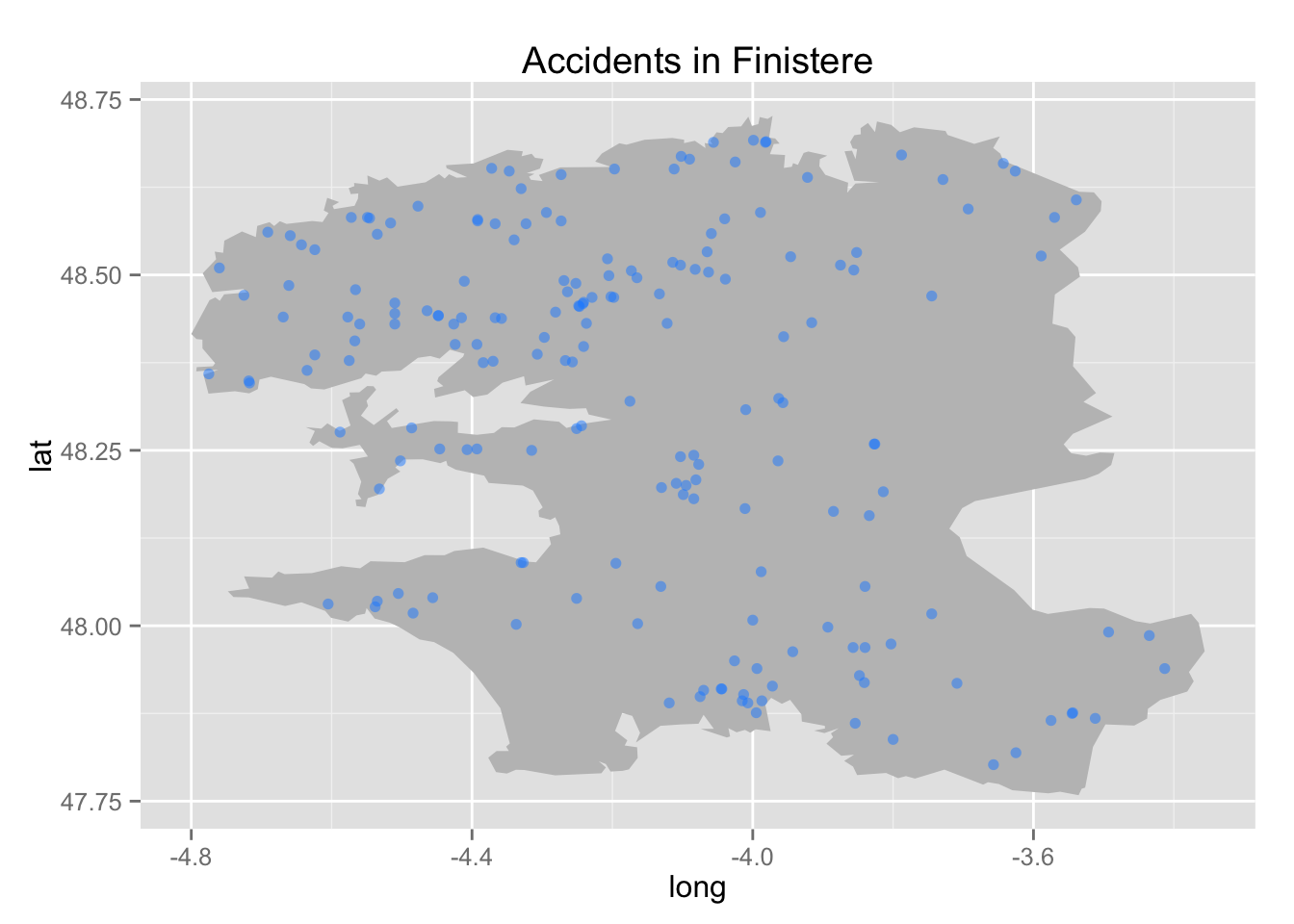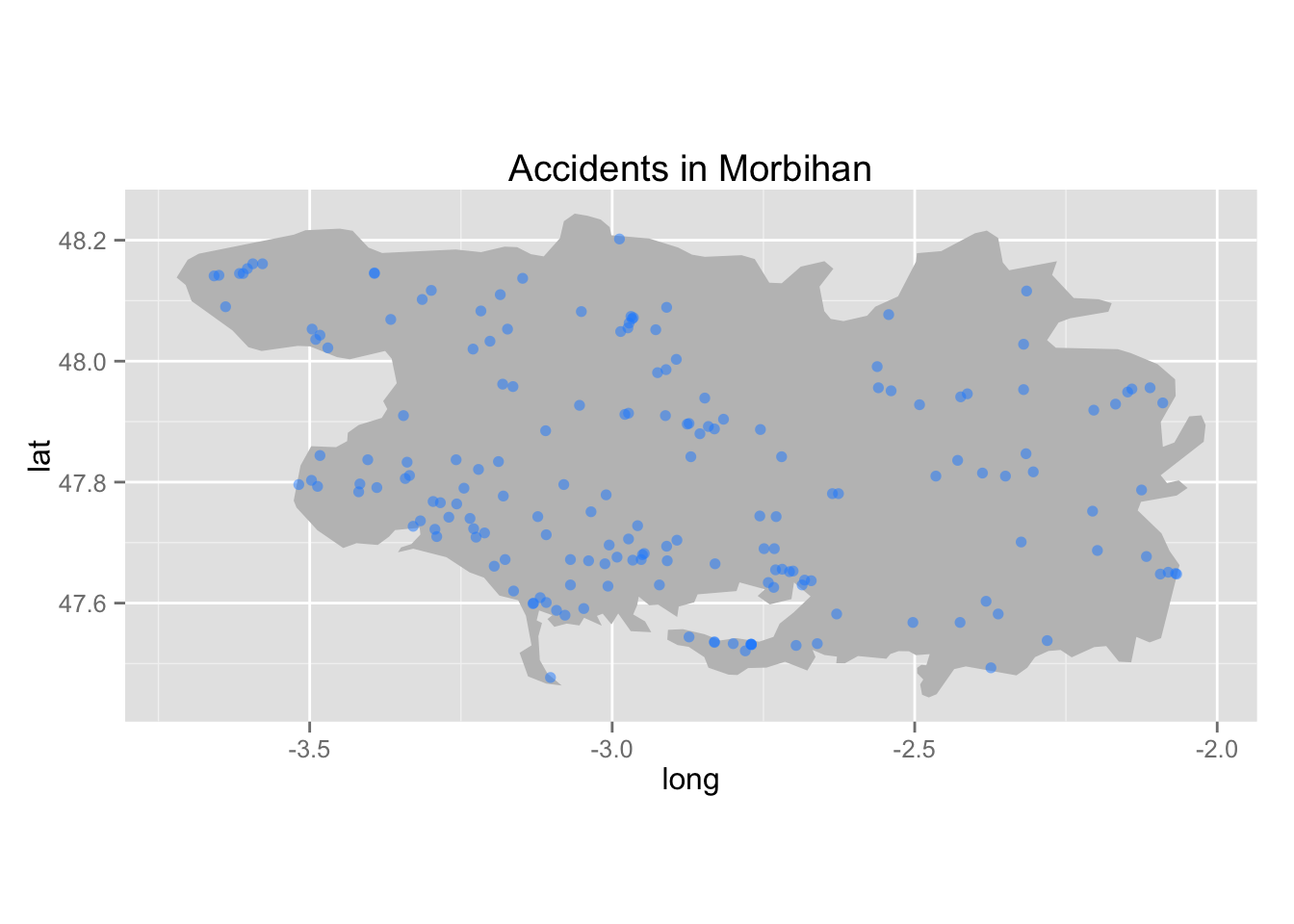This page proposes some R codes to compute the kernel density estimates of two-dimensional data points, using an extension of Ripley’s circumference method to correct for border bias. First, the functions computing the estimates are given. Then, we provide a function to plot the result on a map. And we finish with three examples:

## Density estimation functions

### Help functions

The function `sCircle()` returns `n` points on a circle centered in `centre` with a radius of `radius`.

``````# @n:       number of points to define the circle
# @centre:  center of the circle
sCircle <- function(n = 100, centre = c(0, 0), radius){
theta <- seq(0, 2*pi, length = n)
m <- cbind(cos(theta), sin(theta)) * radius
m[, 1] <- m[, 1] + centre
m[, 2] <- m[, 2] + centre
colnames(m) <- c("x", "y")
m
}# End of sCircle()``````

The function `sWeights()` returns the proportion of the area of a circle of center `x` and radius `1.759*h` on the area of a polygon names `polygon`.

``````# @x:       center of the circle,
# @h:       bandwidth scalar,
# @polygon: polygon on which data points lie.
sWeights <- function(x, h, polygon) {
leCercle <- sCircle(centre = x, radius = 1.759*h)
POLcercle <- as(leCercle[-nrow(leCercle),], "gpc.poly")
return(area.poly(intersect(polygon, POLcercle)) / area.poly(POLcercle))
}# End of sWeights()``````

### Estimation with correction

The function `sKDE()` computes the kernel density estimates, correcting for a possible frontier bias. It returns a list, whose elements are : - `X`: x coordinates at wich estimate is evaluated; - `Y`: y coordinates at wich estimate is evaluated; - `Z`: density estimates; - `ZNA`: density estimates with NA values for points outside the polygon; - `H`: bandwidth matrix; - `W`: vector of weights.

``````# @U:           data points,
# @polygon:     polygon on which points lie,
# @optimal:     if TRUE, uses Hpi() to select the optimal bandwidth,
# @h:           only if optimal=FALSE, scalar bandwidth,
# @parallel:    if TRUE, computes the weights using SNOW clusters,
# @n_clusters:  only if n_clusters=TRUE, defines the number of clusters.
sKDE <- function(U, polygon, optimal = TRUE, h = .1, parallel = FALSE, n_clusters = 4){
if(!class(polygon) == "gpc.poly") polygon <- as(polygon, "gpc.poly")
if(class(U) == "data.frame") U <- as.matrix(U)
IND <- which(is.na(U[, 1]) == FALSE)
U <- U[IND,]
n <- nrow(U)
if(optimal){
H <- Hpi(U, binned = FALSE)
H <- matrix(c(sqrt(H[1, 1] * H[2, 2]), 0, 0, sqrt(H[1, 1] * H[2, 2])), 2, 2)
}
if(!optimal){
H <- matrix(c(h, 0, 0, h), 2, 2)
}

# Help function to compute weights
poidsU <- function(i, U, h, POL){
x <- as.numeric(U[i,])
sWeights(x, h, POL)
}
# Use parallel methods to compute if the number of observation is a bit high
# Change the number of slaves according to the number of cores your processor has
# It is recommended to use a maximum of the number of cores minus one.
if(parallel){
cl <- makeCluster(n_clusters, type = "SOCK")
clusterEvalQ(cl, library("gpclib"))
clusterEvalQ(cl, library("sp"))
clusterExport(cl, c("sCircle", "sWeights"))
OMEGA <- parLapply(cl, 1:n, poidsU, U = U, h = sqrt(H[1, 1]), POL = polygon)
OMEGA <- do.call("c", OMEGA)
stopCluster(cl)
}else{
OMEGA <- NULL
for(i in 1:n){
OMEGA <- c(OMEGA, poidsU(i, U, h = sqrt(H[1, 1]), POL = polygon))
}
}

# Kernel Density Estimator
fhat <- kde(U, H, w = 1/OMEGA,
xmin = c(min(get.bbox(polygon)\$x), min(get.bbox(polygon)\$y)),
xmax = c(max(get.bbox(polygon)\$x), max(get.bbox(polygon)\$y)))
fhat\$estimate <- fhat\$estimate * sum(1/OMEGA) / n

vx <- unlist(fhat\$eval.points)
vy <- unlist(fhat\$eval.points)
VX <- cbind(rep(vx, each = length(vy)))
VY <- cbind(rep(vy, length(vx)))
VXY <- cbind(VX, VY)
Ind <- matrix(inside.gpc.poly(x = VX, y = VY, polyregion = polygon), length(vy), length(vx))
f0 <- fhat
f0\$estimate[t(Ind) == 0] <- NA

list(
X = fhat\$eval.points[],
Y = fhat\$eval.points[],
Z = fhat\$estimate,
ZNA = f0\$estimate,
H = fhat\$H,
W = fhat\$w)
}# End of sKDE()``````

### Estimation without correction

The function `sKDE_without_c()` computes the kernel density estimates, without correcting for a possible frontier bias. It returns a list, whose elements are : - `X`: x coordinates at wich estimate is evaluated; - `Y`: y coordinates at wich estimate is evaluated; - `Z`: density estimates; - `ZNA`: density estimates with NA values for points outside the polygon; - `H`: bandwidth matrix; - `W`: vector of weights.

``````# @U:           data points,
# @polygon:     polygon on which points lie,
# @optimal:     if TRUE, uses Hpi() to select the optimal bandwidth,
# @h:           only if optimal=FALSE, scalar bandwidth,
sKDE_without_c = function(U, polygon, optimal = TRUE, h = .1){
polygon <- as(polygon, "gpc.poly")
IND <- which(is.na(U[,1]) == FALSE)
U <- U[IND,]
n <- nrow(U)
if(optimal){
H <- Hpi(U,binned=FALSE)
H <- matrix(c(sqrt(H[1, 1] * H[2, 2]), 0, 0, sqrt(H[1, 1] * H[2, 2])), 2, 2)
}
if(!optimal){
H <- matrix(c(h, 0, 0, h), 2, 2)
}

# Kernel density estimator
fhat <- kde(U, H,
xmin = c(min(get.bbox(polygon)\$x), min(get.bbox(polygon)\$y)),
xmax = c(max(get.bbox(polygon)\$x), max(get.bbox(polygon)\$y)))

vx <- unlist(fhat\$eval.points)
vy <- unlist(fhat\$eval.points)
VX <- cbind(rep(vx, each = length(vy)))
VY <- cbind(rep(vy, length(vx)))
VXY <- cbind(VX,VY)
Ind <- matrix(inside.gpc.poly(x = VX, y = VY, polyregion = polygon), length(vy), length(vx))
f0 <- fhat
f0\$estimate[t(Ind) == 0] <- NA

list(
X = fhat\$eval.points[],
Y = fhat\$eval.points[],
Z = fhat\$estimate,
ZNA = f0\$estimate,
H = fhat\$H,
W = fhat\$W)
}# End of sKDE_without_c()``````

## Plot

Using the result obtained by the evaluation of the functions `sKDE()` or `sKDE_without_c()`, the function `plot_sKDE()` creates a visualization of the kernel density estimates.

``````# @smooth       : result from sKDE() or sKDE_without_c();
# @breaks       : breaks for the legend (seq(min(smooth\$Z)*.95,max(smooth\$Z)*1.05,length=21) by default);
# @polygon      : polygon on which data points lie;
# @coord        : coordinates (long, lat) of data points;
# @alpha_coords : transparency for data points (.8 by default);
# @size_coords  : size for data points (.8 by default);
# @many_points  : if TRUE, @coord must be the result of condense() (package bigvis). It is helpful when there are too many points to display (FALSE by default);
# @colContour   : colour of the contour of the polygon ("white" by default);
# @colPoints    : colour of the data points ("dodger blue" by default);
# @title        : title (if provided) to give to the plot;
# @contour      : if FALSE, contour are not plotted (TRUE by default);
# @round        : round value for the legend (2 by default);
# @text_size    : text size (22 by default).
plot_sKDE <- function(smooth, breaks, polygon, coord, alpha_coords = .8, size_coords = .8,
many_points = FALSE,
colContour="white",
colPoints="dodger blue", title, contour=TRUE,
round = 2, text_size = 22){

# Get the right format for ggplot2
obtenirMelt <- function(smoothed){
res <- melt(smoothed\$ZNA)
res[,1] <- smoothed\$X[res[,1]]
res[,2] <- smoothed\$Y[res[,2]]
names(res) <- list("X","Y","ZNA")
return(res)
}

smCont <- obtenirMelt(smooth)
if(missing(breaks)) breaks <- seq(min(smooth\$Z)*.95,max(smooth\$Z)*1.05,length=21)
smCont\$colour <- cut(smCont[,"ZNA"],breaks=breaks,labels=round(breaks[-1],digits=round))
smCont\$colour2 <- as.character(cut(smCont[,"ZNA"],breaks=breaks,labels=rev(heat.colors(length(breaks)-1))))

if(is.null(polygon\$group)) polygon\$group <- factor(1)

P <- ggplot() +
geom_polygon(data = polygon,  aes(x = long, y = lat, group = group),
fill = NA, col = "black") +
geom_tile(aes(x = X, y = Y, fill = ZNA),
alpha = .9, data = smCont[!is.na(smCont\$ZNA),], na.rm=TRUE)

lesLabels <- round(breaks,round)
lesIndicesLabels <- floor(seq(1,length(lesLabels),length.out=5)) # Only keep 5 points for the legend values
lesIndicesLabels[length(lesIndicesLabels)] <- length(lesLabels) # Making sure we display the last value
lesLabels <- as.character(lesLabels[lesIndicesLabels])
lesLabels[lesLabels=="0"] <- "0.00"

if(contour) P <- P + geom_contour(data = smCont[!is.na(smCont\$ZNA),],
aes(x = X, y = Y, z = ZNA),
alpha=0.6,  colour = colContour,
breaks = breaks[lesIndicesLabels])
if(many_points){
P <- P + geom_point(data = coord, aes(x = long, y = lat, alpha = .count),
col = "blue", size = size_coords) +
scale_alpha_continuous(guide=FALSE)
}else{
P <- P + geom_point(data = coord[,c("long", "lat")], aes(x = long, y = lat),
alpha = alpha_coords, col = "blue", size = size_coords)
}

if(contour){
ind_level <- which(unlist(lapply(ggplot_build(P)\$data, function(x) "level" %in% colnames(x))))
tmp <- ggplot_build(P)\$data[[ind_level]]
ind <- unlist(lapply(unique(tmp\$piece), function(x){
corresp <- which(tmp\$piece == x)
corresp[round(length(corresp)/2)]
}))
tmp\$level_r <- round(tmp\$level, round)
P <- P + geom_text(aes(label = level_r, z = NULL, x = x, y = y), data=tmp[ind,])
}

P <- P + scale_fill_gradient(name="",low='yellow', high='red',
breaks=breaks[lesIndicesLabels],
limits=range(breaks),labels=lesLabels)

P <- P + theme(axis.text.x=element_blank(),
axis.text.y=element_blank(),
axis.ticks.x=element_blank(),
axis.ticks.y=element_blank(),
axis.title=element_blank(),
text = element_text(size = text_size))

P <- P + geom_polygon(data=polygon, mapping=(aes(x=long, y=lat)),
colour="black", fill=NA)
# Add a title if one was provided
if(!missing(title)) P <- P + ggtitle(title)
P
}``````

## Applications

This page provides three example on how to estimate the density of car accidents that happened in Finistère and Morbihan, two French “départements”, on bike thefts in San Francisco and on camping locations in France. Once the estimates are computed, they are plotted on a map. Two estimations are provided: one using a border correction, and the other ignoring this possible issue.

But before going further, some packages need to be loaded.

``````library(rgdal)
library(surveillance)
library(maptools)
library(ggplot2)
library(plyr)
library(ellipse)
library(fields)
library(gpclib)
library(ks)
library(maps)
library(rgeos)
library(snow)
library(sp)
library(ggmap)
library(reshape2)``````

### Car accidents

#### Data

Data are in the “Data” folder.

``load("../data/car_accidents/acci.RData")``

They are contained in the object names `acci`, which is a list of two elements:

• `finistere`: concerns only the “département” Finistère. It is also a list, whose elements are:
• points: data.frame of data points (long, lat),
• polygon: data.frame of bounding surface - Finistere’s frontier - (long, lat);
• `morbihan`: concerns only the “département” Morbihan. It is also a list, whose elements are:
• points: data.frame of data points (long, lat),
• polygon: data.frame of bounding surface - Finistere’s frontier - (long, lat).
``````# Finistere accidents locations
ggplot() + geom_polygon(data = acci\$finistere\$polygon, aes(x = long, y = lat), fill = "grey75") +
geom_point(data = acci\$finistere\$points, aes(x = long, y = lat), col = "dodger blue", alpha = .5) + coord_equal() +
ggtitle("Accidents in Finistere")````````````# Morbihan accidents location
ggplot() + geom_polygon(data = acci\$morbihan\$polygon, aes(x = long, y = lat), fill = "grey75") +
geom_point(data = acci\$morbihan\$points, aes(x = long, y = lat), col = "dodger blue", alpha = .5) + coord_equal() +
ggtitle("Accidents in Morbihan")``````#### Kernel density estimation

Now, let’s see how to use the functions `sKDE()` and `sKDE_without_c()` to compute the kernel density estimates, taking care of possible border bias, and without considering them respectively.

##### Finistère

Let’s do this for Finistère first.

``````# Estimation with correction
smoothed_fin <- sKDE(U = acci\$finistere\$points, polygon = acci\$finistere\$polygon,
optimal=TRUE, parallel = FALSE)``````
``## Warning: Weights don't sum to sample size - they have been scaled accordingly``
``````# Estimation without correction
smoothed_fin_nc <- sKDE_without_c(U = acci\$finistere\$points, polygon = acci\$finistere\$polygon,
optimal=TRUE)``````

To visualize the estimates, it is possible to use the `plot_sKDE()` function.

First, taking care of the border effects:

``````p_acci_fin <- plot_sKDE(smooth = smoothed_fin,
coord = acci\$finistere\$points,
alpha_coords = .8,
size_coords = 1,
breaks = seq(min(smoothed_fin\$ZNA,
smoothed_fin_nc\$ZNA,na.rm=T)*.95,
max(smoothed_fin\$ZNA,
smoothed_fin_nc\$ZNA,na.rm=T)*1.05,
length=21),
polygon = acci\$finistere\$polygon,
round = 3,
colContour = "black") +
ggtitle("With correction") +
coord_equal()

print(p_acci_fin)``````